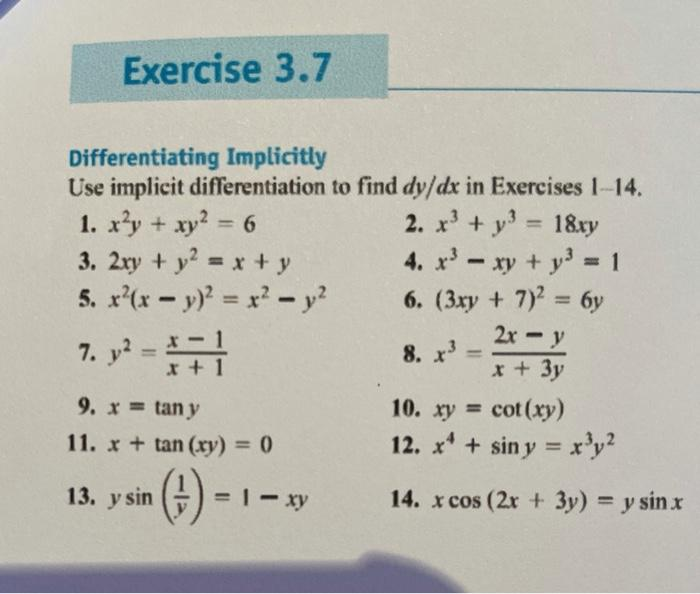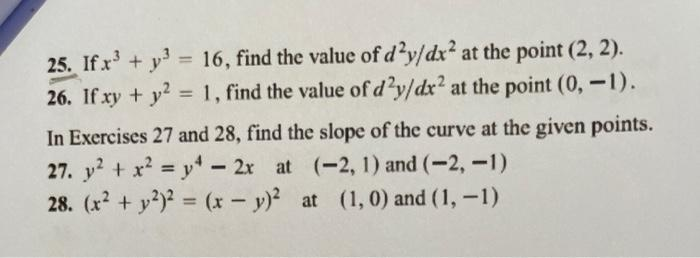### Create an Account

Already have account?

### Forgot Your Password ?

Home / Questions / Exercise 3.7 Differentiating Implicitly Use implicit differentiation to find dy/dx in Exer...

# Exercise 3.7 Differentiating Implicitly Use implicit differentiation to find dy/dx in Exercises 1-14. 1. x²y + xy2 = 6 2. x3 + y3 = 18xy 3. 2xy + y2 = x + y 4. x3 - xy + y) = 1 5. x'(x - y)2 = x2 - y2

Exercise 3.7 Differentiating Implicitly Use implicit differentiation to find dy/dx in Exercises 1-14. 1. x²y + xy2 = 6 2. x3 + y3 = 18xy 3. 2xy + y2 = x + y 4. x3 - xy + y) = 1 5. x'(x - y)2 = x2 - y2 6. (3xy + 7)2 = 6y 7. y2 = 1 X + 1 x + 3y 9. x = tany 10. xy = cot(xy) 11. x + tan (xy) = 0 12. ** + sin y = x 'y? 2x - y 8. x3 13. y sin (1) - = 1 - xy 14. x cos (2x + 3y) = y sin x
25. If.x3 + y = 16, find the value of d’y/dx? at the point (2, 2). 26. If xy + y2 = 1, find the value of dạy/dx? at the point (0, -1). In Exercises 27 and 28, find the slope of the curve at the given points. 27. y2 + x2 = y* - 2x at (-2, 1) and (-2, -1) 28. (x2 + y2)2 = (x - y)2 at (1, 0) and (1, -1)Apr 13 2021 View more View Less

#### Answer (Solved)Subscribe To Get Solution